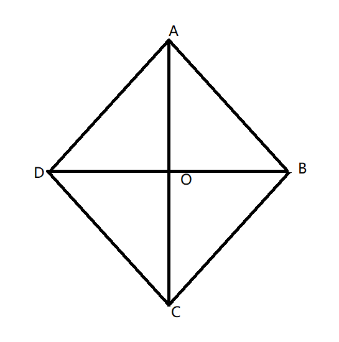QuestionAnswers

# The perimeter of a rhombus is 100 cm. One of its diagonal is 30 cm. Find the length of the other diagonal and the area of the rhombus.Hint: We will first find the length of each side of rhombus from the given perimeter of the rhombus. Use the property of rhombus and given diagonal to find the other diagonal of the rhombus. Hence, calculate the area of the rhombus.

The perimeter of the rhombus is 100 cm.
It is known that all sides of rhombus are equal.
Let the length of side of rhombus be $x$,
Then perimeter of rhombus is $4x$
Thus, $4x = 100 \\ x = 25 \\$
Hence, the length of each side of the rhombus is 25 cm.
We are given that the length of one of its diagonal is 30 cm.In a rhombus, diagonals are perpendicular bisectors
Let diagonals bisect at $O$.
Let $AC$ be 30 cm., then the length of $AO$ is $\dfrac{{30}}{2} = 15$cm
Also, triangle $\Delta AOB$ is a right triangle.
Apply Pythagoras theorem in $\Delta AOB$
$A{B^2} = A{O^2} + O{B^2}$
We have $AB = 25cm$ and $AO = 15cm$,
Therefore,
${\left( {25} \right)^2} = {\left( {15} \right)^2} + O{B^2} \\ O{B^2} = {\left( {25} \right)^2} - {\left( {15} \right)^2} \\$
Simplifying the expression using the formula ${a^2} - {b^2} = \left( {a + b} \right)\left( {a - b} \right)$
$O{B^2} = {\left( {25} \right)^2} - {\left( {15} \right)^2} \\ O{B^2} = \left( {25 + 15} \right)\left( {25 - 15} \right) \\ O{B^2} = 40\left( {10} \right) \\ O{B^2} = 400 \\ OB = 20 \\$
Hence, the length of the other diagonal will be twice the length $OB$, which is 40units.
Next, we will find the area of the rhombus whose formula is $\dfrac{{{d_1} \times {d_2}}}{2}$, where ${d_1}$ and ${d_2}$ are the length of diagonals.
Thus, area of rhombus is,
$\dfrac{{40 \times 30}}{2} = \dfrac{{1200}}{2} = 600c{m^2}$

Note: One must be familiar with the properties of rhombus. Also, in this question, we have used Pythagoras theorem, so one must know how to apply Pythagoras theorem in any right triangle.
View Notes
Area of a Rhombus FormulaPerimeter of a Trapezoid FormulaThe Difference Between an Animal that is A Regulator and One that is A ConformerThe Perimeter of Rectangle FormulaTable of 100 - Multiplication Table of 100What is the full form of phd?The Making of a ScientistChanging the Period of a PendulumCBSE Class 8 Maths Chapter 2 - Linear Equations in One Variable FormulasWhere There is a Will There is a Way EssayCBSE Class 8 Science Reaching The Age of Adolescence WorksheetsImportant Questions for CBSE Class 6 English Honeysuckle Chapter 8 - A Game of ChanceImportant Questions for CBSE Class 6 English A Pact with The Sun Chapter 8 - A Pact with the SunImportant Questions for CBSE Class 8 English Honeydew Chapter 3 - Glimpses of the PastImportant Questions for CBSE Class 8 Science Chapter 10 - Reaching The Age of AdolescenceImportant Questions for CBSE Class 11 Biology Chapter 8 - Cell The Unit of LifeImportant Questions for CBSE Class 8 Social Science Our Past 3 Chapter 4 - Tribals, Dikus and the Vision of a Golden AgeImportant Questions for CBSE Class 12 Maths Chapter 8 - Application of IntegralsImportant Questions for CBSE Class 6 English A Pact with The Sun Chapter 1 - A Tale of Two BirdsImportant Questions for CBSE Class 8 Maths Chapter 2 - Linear Equations in One VariablePrevious Year Question Paper of CBSE Class 10 EnglishCBSE Class 10 Hindi A Question Paper 2020Hindi A Class 10 CBSE Question Paper 2009Hindi A Class 10 CBSE Question Paper 2015Hindi A Class 10 CBSE Question Paper 2007Hindi A Class 10 CBSE Question Paper 2013Hindi A Class 10 CBSE Question Paper 2016Hindi A Class 10 CBSE Question Paper 2012Hindi A Class 10 CBSE Question Paper 2010Hindi A Class 10 CBSE Question Paper 2008RS Aggarwal Class 8 Mathematics Solutions for Chapter-18 Area of a Trapezium and a PolygonCBSE Class 8 Maths Chapter 2 Linear Equation in One Variable Exercise 2.5NCERT Solutions for Class 9 English Moments Chapter 8 - A House is Not a HomeNCERT Solutions for Class 8 Maths Chapter 2 Linear Equations in One VariableReaching The Age of Adolescence NCERT Solutions - Class 8 ScienceReaching The Age of Adolescence NCERT Solutions - Class 8 ScienceRS Aggarwal Class 10 Solutions - Perimeter and Areas of Plane FiguresNCERT Solutions for Class 6 English Honeysuckle Chapter 8 - A Game of ChanceRS Aggarwal Solutions Class 8 Chapter-18 Area of a Trapezium and a Polygon (Ex 18C) Exercise 18.3RS Aggarwal Solutions Class 8 Chapter-18 Area of a Trapezium and a Polygon (Ex 18B) Exercise 18.2